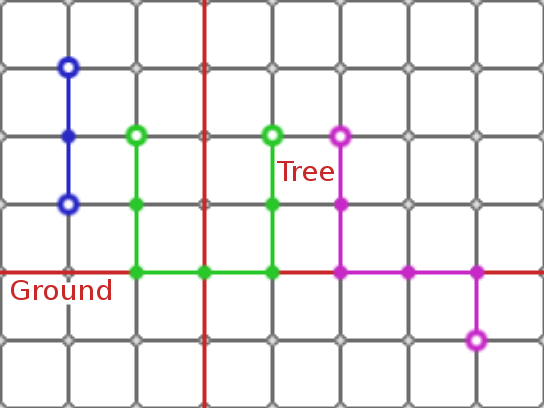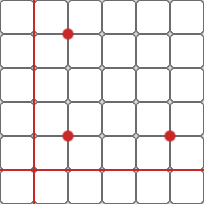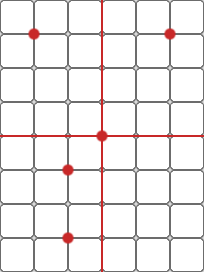### Problem Statement

Monkeys live in trees. Their world can be abstracted as a two-dimensional grid with integer coordinates. The ground is the x-axis and trees are planted perpendicular to the x-axis (in both the positive and negative y directions). Monkeys can only move up and down trees, and horizontally on the ground. Therefore, the distance between two monkeys is calculated as follows:

- If the two monkeys have the same x coordinate (meaning that they're in the same tree), the distance between them is the absolute difference between their y coordinates. See the blue pair in the image below.

- Otherwise, the distance is the distance between monkey1 and the ground + the distance between monkey2 and the ground + the absolute difference between their x coordinates. See the green and purple pairs in the image below.The government is about to make a bold decision that will make the lives of the monkeys easier. They will minimize the maximum distance between any pair of monkeys by moving the ground to a horizontal line y = N, where N is an integer. If the ground is already at the optimal position, it will not be moved.

You are given two int[]s x and y, The ith monkey lives at (x[i], y[i]), where the absolute values of x[i] and y[i] are each less than or equal to 109. Return the maximum distance between any pair of monkeys after the the ground is moved to the optimal position. The distance should be returned as a String with no leading zeros.

### Definition

 Class: MonkeyTreeDistance Method: minimalMaximumDistance Parameters: int[], int[] Returns: String Method signature: String minimalMaximumDistance(int[] x, int[] y) (be sure your method is public)

### Constraints

-x and y will each contain between 2 and 50 elements, inclusive.
-x and y will contain the same number of elements.
-Neither two monkeys will occupy the same position.
-The absolute value of each element in x and y will be between 0 and 10^9, inclusive.

### Examples

0)

 `{1, 4, 1}` `{4, 1, 1}`
`Returns: "6"`1)

 `{-1, -2, -1, 0, 2}` `{-1, 3, -3, 0, 3}`
`Returns: "9"`2)

 `{1, 1, 1, 1}` `{1, 10, 100, 1000}`
`Returns: "999"`
 These four monkeys live in the same tree, so moving the ground will not affect any of the distances.

#### Problem url:

http://www.topcoder.com/stat?c=problem_statement&pm=8020

#### Problem stats url:

http://www.topcoder.com/tc?module=ProblemDetail&rd=10790&pm=8020

mohamedafattah

#### Testers:

PabloGilberto , brett1479 , Olexiy , ivan_metelsky

#### Problem categories:

Greedy, Math, Search